Home MathLab Plotting in Matlab – The Basics

# Plotting in Matlab – The Basics

We have recently used the EZPLOT technique to plot in Matlab, which is indeed an easier technique to plot compared to the one we are going to make use of in this post.

Plotting functions gives us a visual description of the behavior of the latter as we change the system variable. And Matlab is THE TOOLS that will help you plot with the less hassle possible while giving you a wide range of handiness of what you will be capable of.

## Plotting in Matlab

plot(A,B) plots vector B versus vector A and plot(Y) plots the columns of Y versus their index.

### Plotting example 1

Let’s plot one of the polynomial equations we have recently solved here.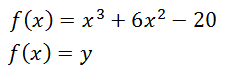Plotting this function in Matlab will look like the following

We first have to select the range of the variable and the incremental value of the variable vector

Here is the code

```x=-20:0.05:20;
y=x.^3+6*x.^2-20;
plot(x,y);
grid on;```

The first line simply means that we want the variable x to start at -20 and to end at 20, and it has to increase with a step of 0.05 from its initial value to its final value. If you type the following code in Matlab (without the; at the end)

`x=-20:0.05:20`

Matlab will give you all the values of the element of the vector x.

The Second line tells Matlab to compute y for each value of x.

Grid on asks Matlab to display the grid while plotting y versus x.

Here is the plot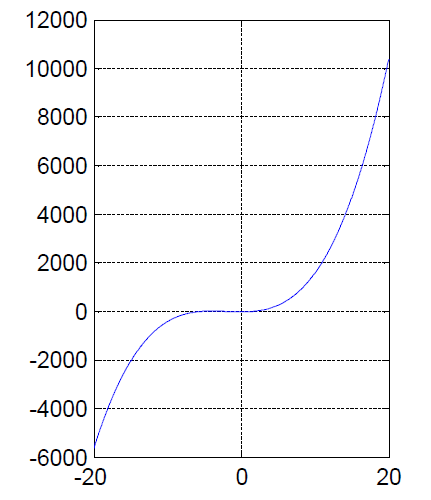### Plotting example 2

In this example, let’s plot 2 graphs in one plot. The following are the functions: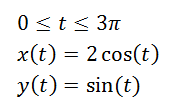Here is the code

```t=0:pi/50:3*pi;
xt=2*cos(t);
yt=sin(t);
plot(t,xt,'r',t,yt,'b');
grid on;```

And the plot is the following.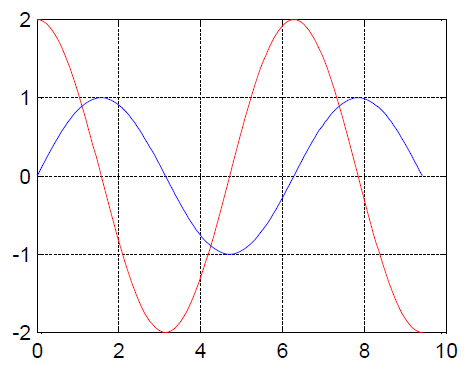The following line

`plot(t,xt,'r',t,yt,'b');`

Means that I want x(t) plot to be Red and y(t) plot to be Blue.

Use the line

`Help PLot`

or

`Doc plot`

### Plotting example 3

Let’s plot a circle of 0.5 of radius

code:

```Alpha=linspace(0,2*pi);
plot(0.5*cos(Alpha), 0.5*sin(Alpha),'LineWidth',2)```

The plot: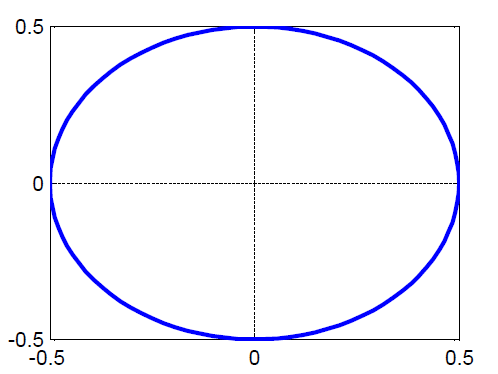‘LineWidth’,2 simply set the thickness of the plot to 2.

linspace(A, B) generates a row vector of 100 linearly equally spaced points between A and B

Here are just the basics in plotting with Matlab, but with what you have just learned you can start plotting and always remember that you can use Matlab Help to learn more while using Matlab.